Next: Retrieve domain properties Up: Elements of the Fib Previous: Call external objects   Contents   Index

## External subobjects

External subobjects are objects, that are already provided during the evaluation of the current Fib object. (See the subobjects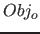in section 9.9 on page)

Syntax: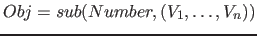Description of the elements:

•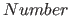: The number of the external subobject (corresponding to an output variable vector in the next root-element), which is to be used here. Thecan only be fixed integer value. The domain of theis implicit in the number of external subobjects (or output variable vectors) of the next root-element (the root-element, in which the subelement appears in the main-Fib-object), see section 9.14 on page. If the value of the specified number is outside the valid domain (this is equivalent to an error), it is rounded to the next value, which lies in the domain.
•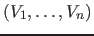: The vector with the output values, which are needed for the used Fib object.
•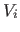: This are the output values, which are needed for the used Fib object. For them the output variables (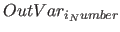) in the im external object element (obj) are defined. The output values have the same number and order as the'th output variables in the corresponding external object element (obj). Nevertheless, if the number does not match (equivalent to a faulty Fib object), the variables that are too much are ignored, and for the missing variables, the zero value of the domain of the corresponding output variable in the root-element is used.

Notes: With external subobjects multimedia information can be provided for the Fib object, which can be used in certain places. This is useful for example, if the current Fib object, implements a calculation or function, which is more extensive and is required several times in other Fib objects.

If an external subobject dosn't exists for the evaluation of a root element, an empty point(point without impact) will be used for it. external subobjects can even be useful for the top most root-element, if for example the multimedia object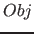is just a frame, in which an other picture can be displayed from an given start point (given by the output values).

Examples:

•: At this point, the first external subobject of the next root-element is used.
•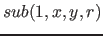: At this point, the first external subobject of the next root-element is used. The variables,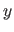and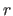are provided for the external subobject. These variables could for example be used for the position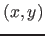andfor the amount of red of the point, which is used for the external subobject.Next: Retrieve domain properties Up: Elements of the Fib Previous: Call external objects   Contents   Index
Betti Österholz 2013-02-13